# IPCC AR5 Chapter 12 (selected figures)¶

## Overview¶

The goal is to create a standard recipe for creating selected Figures from IPCC AR5 Chapter 12 on “Long-term Climate Change: Projections, Commitments and Irreversibility”. These include figures showing the change in a variable between historical and future periods, e.g. maps (2D variables), zonal means (3D variables), timeseries showing the change in certain variables from historical to future periods for multiple scenarios, and maps visualizing change in variables normalized by global mean temperature change (pattern scaling) as in Collins et al., 2013.

## Available recipes and diagnostics¶

Recipes are stored in recipes/

• recipe_collins13ipcc.yml

Diagnostics are stored in diag_scripts/

• ipcc_ar5/ch12_map_diff_each_model_fig12-9.ncl: calculates the difference between future and historical runs for one scenario for each given model individually on their native grid and plots all of them in one Figure. As in Figure 12.9 in AR5.

• ipcc_ar5/ch12_ts_line_mean_spread.ncl: calculates time series for one variable, change in future relative to base period in historical, multi-model mean as well as spread around it (as standard deviation).

• ipcc_ar5/ch12_plot_ts_line_mean_spread.ncl: plots the timeseries multi-model mean and spread calculated above. As in Figure 12.5 in AR5.

• ipcc_ar5/ch12_calc_IAV_for_stippandhatch.ncl: calculates the interannual variability over piControl runs, either over the whole time period or in chunks over some years.

• ipcc_ar5/ch12_calc_map_diff_mmm_stippandhatch.ncl: calculates the difference between future and historical periods for each given model and then calculates multi-model mean as well as significance. Significant is where the multi-model mean change is greater than two standard deviations of the internal variability and where at least 90% of the models agree on the sign of change. Not significant is where the multi-model mean change is less than one standard deviation of internal variability.

• ipcc_ar5/ch12_plot_map_diff_mmm_stipp.ncl: plots multi-model mean maps calculated above including stippling where significant and hatching where not significant. As in Figure 12.11 in AR5.

• ipcc_ar5/ch12_calc_zonal_cont_diff_mmm_stippandhatch.ncl: calculates zonal means and the difference between future and historical periods for each given model and then calculates multi-model mean as well as significance as above.

• ipcc_ar5/ch12_plot_zonal_diff_mmm_stipp.ncl: plots the multi-model mean zonal plots calculated above including stippling where significant and hatching where not significant. As in Figure 12.12 in AR5.

• ipcc_ar5/ch12_calc_map_diff_scaleT_mmm_stipp.ncl: calculates the change in variable between future and historical period normalized by gloabl mean temperature change of each given model and scenario. Then averages over all realizations and calculates significance. Significant is where the mean change averaged over all realizations is larger than the 95% percentile of the distribution of models (assumed to be gaussian). Can be plotted using ipcc_ar5/ch12_plot_map_diff_mmm_stipp.ncl.

• seaice/seaice_ecs.ncl: scatter plot of historical trend in September Arctic sea ice extent (SSIE) vs historical long-term mean SSIE (similar to Fig. 12.31a in AR5) and historical SSIE trend vs YOD RCP8.5 (similar to Fig. 12.31d in AR5).

• seaice/seaice_yod.ncl: calculation of year of near disappearance of Arctic sea ice (similar to Fig 12.31e in AR5)

• ipcc_ar5/ch12_snw_area_change_fig12-32.ncl: calculate snow area extent in a region (e.g Northern Hemisphere) and season (e.g. Northern Hemisphere spring March & April) relative to a reference period (e.g 1986-2005) and spread over models as in Fig. 12.32 of IPCC AR5. Can be plotted using ipcc_ar5/ch12_plot_ts_line_mean_spread.ncl.

## User settings¶

1. Script ipcc_ar5/ch12_map_diff_each_model_fig12-9.ncl

Required settings (script)

• time_avg: time averaging (“annualclim”, “seasonalclim”)

• experiment: IPCC Scenario, used to pair historical and rcp runs from same model

Optional settings (script)

• projection: map projection, any valid ncl projection, default = Robinson

• max_vert: maximum number of plots in vertical

• max_hori: maximum number of plots in horizontal

• title: plot title

• colormap: alternative colormap, path to rgb file or ncl name

• diff_levs: list with contour levels for plots

• span: span whole colormap? (True, False, default = False)

Required settings (variables)

• project: CMIP5 (or CMIP6)

• mip: variable mip, generally Amon or Omon

Required settings (script)

• scenarios: list with scenarios included in figure

• syears: list with start years in time periods (e.g. start of historical period and rcps)

• eyears: list with end years in time periods (end year of historical runs and rcps)

• begin_ref_year: start year of reference period (e.g. 1986)

• end_ref_year: end year of reference period (e.g 2005)

• label: list with labels to use in legend depending on scenarios

Optional settings (script)

• spread: how many standard deviations to calculate the spread with? default is 1., ipcc tas used 1.64

• model_nr: save number of model runs per period and scenario in netcdf to print in plot? (True, False, default = False)

• ts_minlat: minimum latitude if not global

• ts_maxlat: maximum latitude if not global

• ts_minlon: minimum longitude if not global

• ts_maxlon: maximum longitude if not global

Required settings (variables)

• project: CMIP5 (or CMIP6)

• mip: variable mip, generally Amon or Omon

Required settings (script)

• ancestors: variable and diagnostics that calculated data to be plotted

Optional settings (script)

• title: specify plot title

• yaxis: specify y-axis title

• ymin: minimim value on y-axis, default calculated from data

• ymax: maximum value on y-axis

• colormap: alternative colormap, path to rgb file or ncl name

4. Script ipcc_ar5/ch12_calc_IAV_for_stippandhatch.ncl:

Required settings (script)

• time_avg: time averaging (“annualclim”, “seasonalclim”), needs to be consistent with calculation in ch12_calc_map_diff_mmm_stippandhatch.ncl

Optional settings (script)

• periodlength: length of period in years to calculate variability over, default is total time period

• iavmode: calculate IAV from multi-model mean or save individual models (“each”: save individual models, “mmm”: multi-model mean, default), needs to be consistent with ch12_calc_map_diff_mmm_stippandhatch.ncl

Required settings (variables)

• project: CMIP5 (or CMIP6)

• mip: variable mip, generally Amon or Omon

• exp: piControl

• preprocessor: which preprocessor to use, depends on dimension of variable, for 2D preprocessor only needs to regrid, for 3D we need to extract levels either based on reference_dataset or specify levels.

Optional settings (variables)

• reference_dataset: the reference dataset for level extraction in case of 3D variables.

5. Script ipcc_ar5/ch12_calc_map_diff_mmm_stippandhatch.ncl:

Required settings (script)

• ancestors: variable and diagnostics that calculated interannual variability for stippling and hatching

• time_avg: time averaging (“annualclim”, “seasonalclim”)

• scenarios: list with scenarios to be included

• periods: list with start years of periods to be included

• label: list with labels to use in legend depending on scenarios

Optional settings (script)

• seasons: list with seasons index if time_avg “seasonalclim” (then required), DJF:0, MAM:1, JJA:2, SON:3

• iavmode: calculate IAV from multi-model mean or save individual models (“each”: save individual models, “mmm”: multi-model mean, default), needs to be consistent with ch12_calc_IAV_for_stippandhatch.ncl

• percent: determines if difference expressed in percent (0, 1, default = 0)

Required settings (variables)

• project: CMIP5 (or CMIP6)

• mip: variable mip, generally Amon or Omon

• preprocessor: which preprocessor to use, preprocessor only needs to regrid

6. Script ipcc_ar5/ch12_plot_map_diff_mmm_stipp.ncl:

Required settings (script)

• ancestors: variable and diagnostics that calculated field to be plotted

Optional settings (script)

• projection: map projection, any valid ncl projection, default = Robinson

• diff_levs: list with explicit levels for all contour plots

• max_vert: maximum number of plots in vertical

• max_hori: maximum number of plots in horizontal

• model_nr: save number of model runs per period and scenario in netcdf to print in plot? (True, False, default = False)

• colormap: alternative colormap, path to rgb file or ncl name

• span: span whole colormap? (True, False, default = True)

• sig: plot stippling for significance? (True, False)

• not_sig: plot hatching for uncertainty? (True, False)

• pltname: alternative name for output plot, default is diagnostic + varname + time_avg

• units: units written next to colorbar, e.g (~F35~J~F~C)

7. Script ipcc_ar5/ch12_calc_zonal_cont_diff_mmm_stippandhatch.ncl:

Required settings (script)

• ancestors: variable and diagnostics that calculated interannual variability for stippling and hatching

• time_avg: time averaging (“annualclim”, “seasonalclim”)

• scenarios: list with scenarios to be included

• periods: list with start years of periods to be included

• label: list with labels to use in legend depending on scenarios

Optional settings (script)

• base_cn: if want contours of base period as contour lines, need to save base period field (True, False)

• seasons: list with seasons index if time_avg “seasonalclim” (then required), DJF:0, MAM:1, JJA:2, SON:3

• iavmode: calculate IAV from multi-model mean or save individual models (“each”: save individual models, “mmm”: multi-model mean, default), needs to be consistent with ch12_calc_IAV_for_stippandhatch.ncl

• percent: determines if difference expressed in percent (0, 1, default = 0)

Required settings (variables)

• project: CMIP5 (or CMIP6)

• mip: variable mip, generally Amon or Omon

• preprocessor: which preprocessor to use, preprocessor needs to regrid, extract leves and calculate the zonal mean.

Optional settings (variables)

• reference_dataset: the reference dataset for level extraction

8. Script ipcc_ar5/ch12_plot_zonal_diff_mmm_stipp.ncl:

Required settings (script)

• ancestors: variable and diagnostics that calculated field to be plotted

Optional settings (script)

• diff_levs: list with explicit levels for all contour plots

• max_vert: maximum number of plots in vertical

• max_hori: maximum number of plots in horizontal

• model_nr: save number of model runs per period and scenario in netcdf to print in plot? (True, False, default = False)

• colormap: alternative colormap, path to rgb file or ncl name

• span: span whole colormap? (True, False, default = True)

• sig: plot stippling for significance? (True, False)

• not_sig: plot hatching for uncertainty? (True, False)

• pltname: alternative name for output plot, default is diagnostic + varname + time_avg

• units: units written next to colorbar in ncl strings, e.g (m s~S~-1~N~)

• if base_cn: True in ch12_calc_zonal_cont_diff_mmm_stippandhatch.ncl further settings to control contour lines:

• base_cnLevelSpacing: spacing between contour levels

• base_cnMinLevel: minimum contour line

• base_cnMaxLevel: maximum contour line

9. Script ipcc_ar5/ch12_calc_map_diff_scaleT_mmm_stipp.ncl:

Required settings (script)

• time_avg: time averaging (“annualclim”, “seasonalclim”)

• scenarios: list with scenarios to be included

• periods: list with start years of periods to be included

• label: list with labels to use in legend depending on scenarios

Optional settings (script)

• seasons: list with seasons index if time_avg “seasonalclim” (then required), DJF:0, MAM:1, JJA:2, SON:3

• percent: determines if difference expressed in percent (0, 1, default = 0)

Required settings (variables)

• project: CMIP5 (or CMIP6)

• mip: variable mip, generally Amon or Omon

• preprocessor: which preprocessor to use, preprocessor only needs to regrid

10. Script ipcc_ar5/ch12_snw_area_change_fig12-32.ncl:

Required settings (script)

• scenarios: list with scenarios included in figure

• syears: list with start years in time periods (e.g. start of historical period and rcps)

• eyears: list with end years in time periods (end year of historical runs and rcps)

• begin_ref_year: start year of reference period (e.g. 1986)

• end_ref_year: end year of reference period (e.g 2005)

• months: first letters of months included in analysis? e.g. for MA (March + April) for Northern Hemisphere

• label: list with labels to use in legend depending on scenarios

Optional settings (script)

• spread: how many standard deviations to calculate the spread with? default is 1., ipcc tas used 1.64

• model_nr: save number of model runs per period and scenario in netcdf to print in plot? (True, False, default = False)

• colormap: alternative colormap, path to rgb file or ncl name

• ts_minlat: minimum latitude if not global

• ts_maxlat: maximum latitude if not global

• ts_minlon: minimum longitude if not global

• ts_maxlon: maximum longitude if not global

Required settings (variables)

• project: CMIP5 (or CMIP6)

• mip: variable mip, LImon

• fx_files: [sftlf, sftgif]

11. Script seaice/seaice_ecs.ncl

Required settings (scripts)

• hist_exp: name of historical experiment (string)

• month: selected month (1, 2, …, 12) or annual mean (“A”)

• rcp_exp: name of RCP experiment (string)

• region: region to be analyzed ( “Arctic” or “Antarctic”)

Optional settings (scripts)

• fill_pole_hole: fill observational hole at North pole (default: False)

• styleset: color style (e.g. “CMIP5”)

Optional settings (variables)

• reference_dataset: reference dataset

12. Script seaice/seaice_yod.ncl

Required settings (scripts)

• month: selected month (1, 2, …, 12) or annual mean (“A”)

• region: region to be analyzed ( “Arctic” or “Antarctic”)

Optional settings (scripts)

• fill_pole_hole: fill observational hole at North pole, Default: False

• wgt_file: netCDF containing pre-determined model weights

Optional settings (variables)

• ref_model: array of references plotted as vertical lines

## Variables¶

Note: These are the variables tested and used in IPCC AR5. However, the code is flexible and in theory other variables of the same kind can be used.

• areacello (fx, longitude latitude)

• clt (atmos, monthly mean, longitude latitude time)

• evspsbl (atmos, monthly mean, longitude latitude time)

• hurs (atmos, monthly mean, longitude latitude time)

• mrro (land, monthly mean, longitude latitude time)

• mrsos (land, monthly mean, longitude latitude time)

• pr (atmos, monthly mean, longitude latitude time)

• psl (atmos, monthly mean, longitude latitude time)

• rlut, rsut, rtmt (atmos, monthly mean, longitude latitude time)

• sic (ocean-ice, monthly mean, longitude latitude time)

• snw (land, monthly mean, longitude latitude time)

• sos (ocean, monthly mean, longitude latitude time)

• ta (atmos, monthly mean, longitude latitude lev time)

• tas (atmos, monthly mean, longitude latitude time)

• thetao (ocean, monthly mean, longitude latitude lev time)

• ua (atmos, monthly mean, longitude latitude lev time)

## Reference¶

• Collins, M., R. Knutti, J. Arblaster, J.-L. Dufresne, T. Fichefet, P. Friedlingstein, X. Gao, W.J. Gutowski, T. Johns, G. Krinner, M. Shongwe, C. Tebaldi, A.J. Weaver and M. Wehner, 2013: Long-term Climate Change: Projections, Commitments and Irreversibility. In: Climate Change 2013: The Physical Science Basis. Contribution of Working Group I to the Fifth Assessment Report of the Intergovernmental Panel on Climate Change [Stocker, T.F., D. Qin, G.-K. Plattner, M. Tignor, S.K. Allen, J. Boschung, A. Nauels, Y. Xia, V. Bex and P.M. Midgley (eds.)]. Cambridge University Press, Cambridge, United Kingdom and New York, NY, USA.

## Example plots¶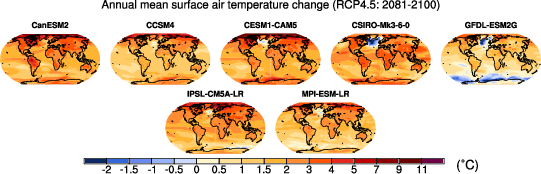Fig. 123 Surface air temperature change in 2081–2100 displayed as anomalies with respect to 1986–2005 for RCP4.5 from individual CMIP5 models.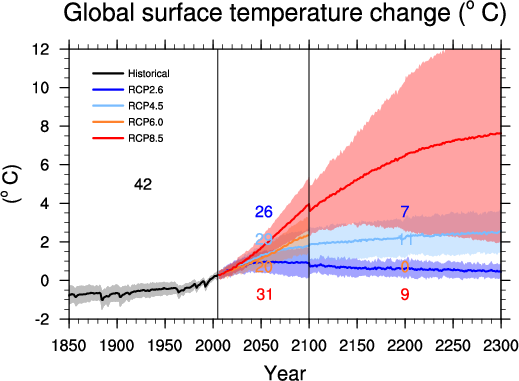Fig. 124 Time series of global annual mean surface air temperature anomalie (relative to 1986–2005) from CMIP5 concentration-driven experiments.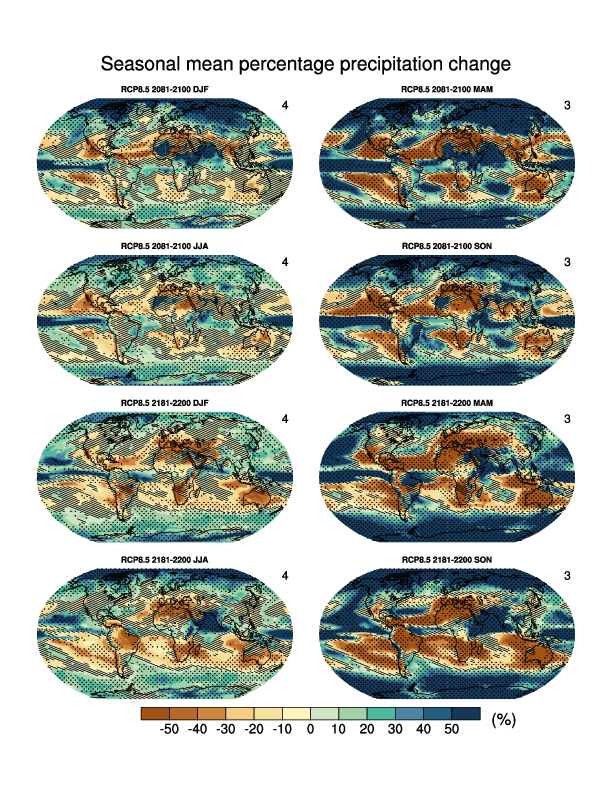Fig. 125 Multi-model CMIP5 average percentage change in seasonal mean precipitation relative to the reference period 1986–2005 averaged over the periods 2081–2100 and 2181–2200 under the RCP8.5 forcing scenario. Hatching indicates regions where the multi-model mean change is less than one standard deviation of internal variability. Stippling indicates regions where the multi-model mean change is greater than two standard deviations of internal variability and where at least 90% of models agree on the sign of change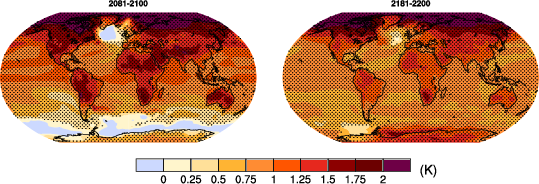Fig. 126 Temperature change patterns scaled to 1°C of global mean surface temperature change.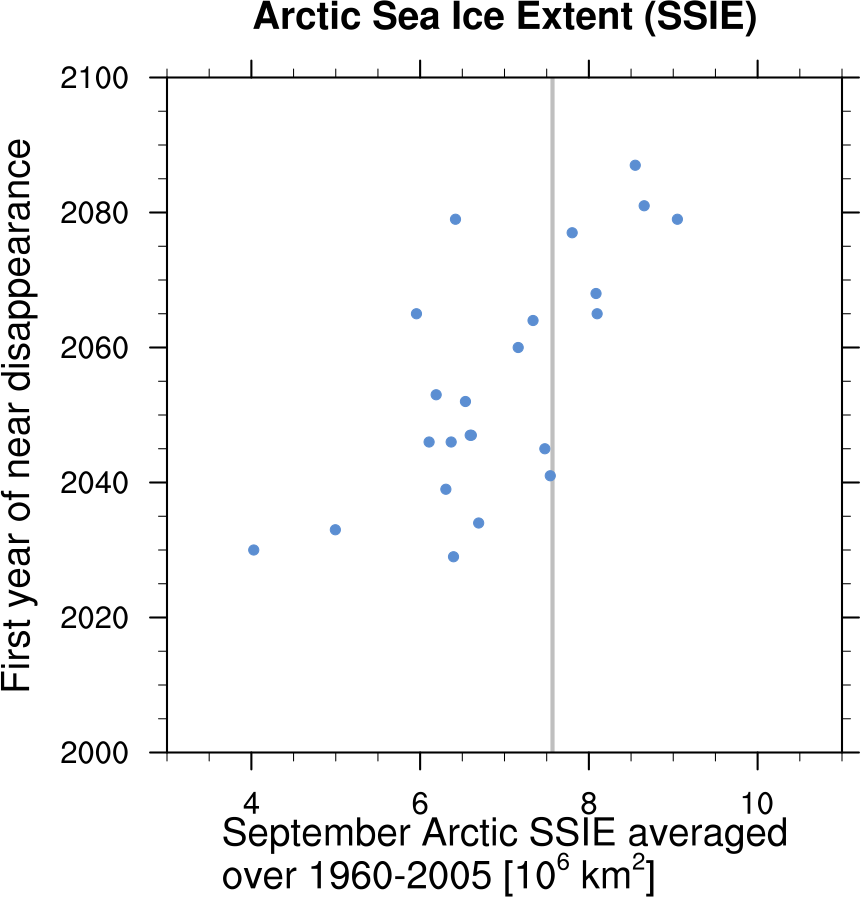Fig. 127 Scatter plot of mean historical September Arctic sea ice extent vs 1st year of disappearance (RCP8.5) (similar to IPCC AR5 Chapter 12, Fig. 12.31a).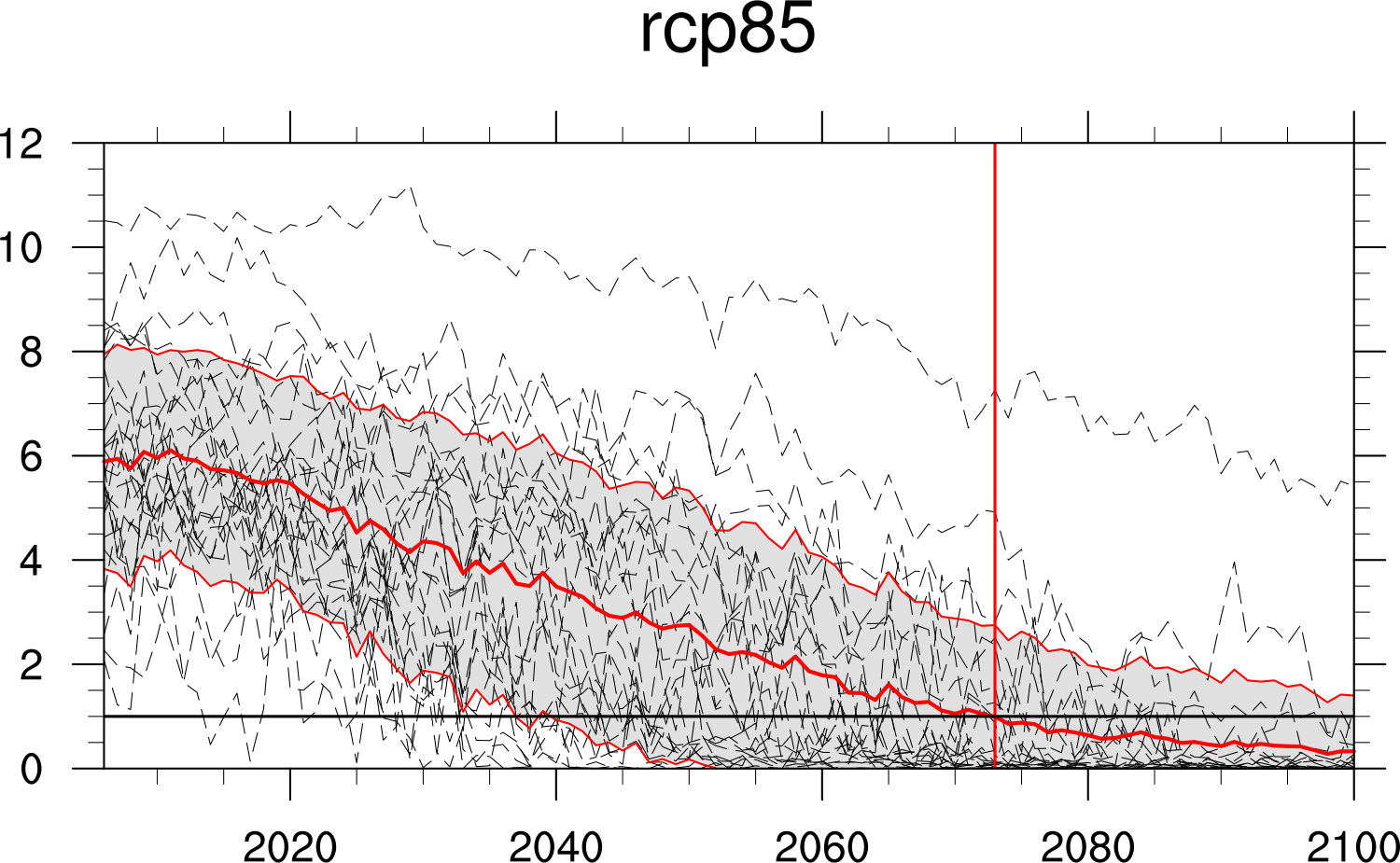Fig. 128 Time series of September Arctic sea ice extent for individual CMIP5 models, multi-model mean and multi-model standard deviation, year of disappearance (similar to IPCC AR5 Chapter 12, Fig. 12.31e).